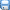## 《有理数的加减混合运算》同步练习1（鲁教版六年级上）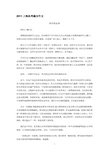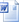资料类别:  数学/同步 所属版本:  鲁教版 所属地区:  全国 上传时间:  2011/7/12 下载次数:  119 次 资料类型: 上传人:  WmiW****@163.com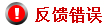### 资料概述与简介

2.6有理数的加减混合运算 1.计算： (1)23－17－(－7)+(－16) (2)+(－)－1+ (3)(－26.54)+(－6.4)－18.54+6.4 (4)(－4)－(－5)+(－4)－3 (5)0+1－［(－1)－(－)－(+5)－(－)］+｜－4｜ 2.有一架直升飞机从海拔1000米的高原上起飞，第一次上升了1500米，第二次上升上－1200米，第三次上升了1100米，第四次上升了－1700米，求此时这架飞机离海平面多少米？ 3.10名学生体检测体重，以50千克为基准，超过的数记为正，不足的数记为负，称得结果如下(单位：千克)： 2,3,－7.5,－3,5,－8,3.5,4.5,8,－1.5 这10名学生的总体重为多少？10名学生的平均体重为多少？ 答案： 1.解：(1)原式=23－17+7－16 =23+7－17－16=30－33=－3 (2)原式=(+－1)+(－)=－ (3)原式=(－26.54)－18.54+［(－6.4)+6.4］=(－26.54)－18.54=－45.08 (4)原式=(－4)+5+(－4)－3=(－4－4－3)+5 =－12+5=－6 (5)原式=1－［(－1)+－5+］+4 =1－［(－1+)－5］+4 =1－(－5)+4=10 2.解：1000+1500+(－1200)+1100+(－1700) =1000+1500－1200+1100－1700 =1000+1500+1100－1200－1700 =3600－2900=700(米) 因此，这时这架飞机离海平面700米. 3.解：2+3+(－7.5)+(－3)+5+(－8)+3.5+4.5+8+(－1.5) =2+3－7.5－3+5－8+3.5+4.5+8－1.5=2+5+3.5+4.5+3－3－8+8－7.5－1.5=6. 因此，10名学生的总体重为： 50×10+6=506(千克) 10名学生的平均体重为： 506÷10=50.6(千克) 初中学习网－中国最大初中学习网站CzxxW.com | 我们负责传递知识！

## 更多>>其他相关资源### 资料ID：

/ /

…下载本资料需要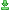下载次